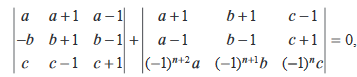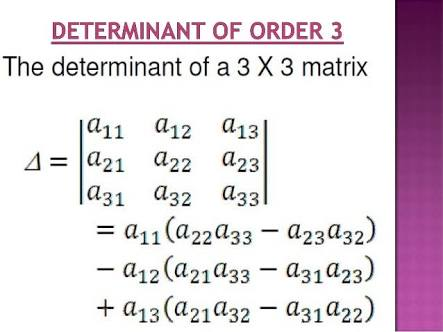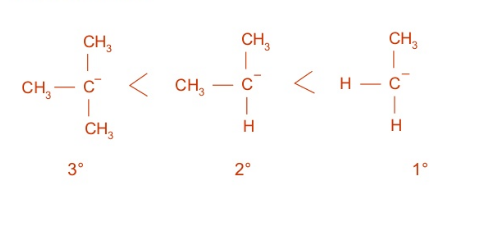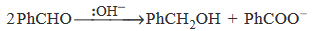## Filters

Sort by :
Q
Engineering
111 Views   |

Let A and B denote the statements

If then

• Option 1)

A is false and B is true

• Option 2)

Both A and B are true

• Option 3)

Both A and B are false

• Option 4)

A is true and B is false

As we learnt in   Addition Formulae - - wherein A and B are two angles.     Adding   to equation, we get              This is only true if  Hence both statements true. Option 1) A is false and B is true This option is incorrect. Option 2) Both A and B are true This option is correct. Option 3) Both A and B are false This option is incorrect. Option 4) A is true and B is false This...
Engineering
125 Views   |

If  are non-coplanar vectors and p,q,are real number, then the equality,

hold for

• Option 1)

exactly two values of

• Option 2)

more than two but not all values of

• Option 3)

all values of

• Option 4)

exactly one value of

As we learnt in  Scalar Triple Product - - wherein Scalar Triple Product of three vectors .     But Option 1) exactly two values of  This option is incorrect. Option 2) more than two but not all values of This option is incorrect. Option 3) all values of  This option is incorrect. Option 4) exactly one value of  This option is correct.
Engineering
170 Views   |

The projections of a vector on the three coordinate axis are 6, -3, 2 respectively. The direction cosines  of the vector are

• Option 1)

• Option 2)

• Option 3)

• Option 4)

As we learnt in  Direction Cosines of a Vector -  are the Direction Cosines. They are denoted by l, m, n,  - wherein    Projections are (6,-3,2) Magnitude = Option 1) This solution is incorrect  Option 2) This solution is correct  Option 3) This solution is incorrect  Option 4) This solution is incorrect
Engineering
104 Views   |

The differential equation which represents the family of curves where are arbitrary constants, is

• Option 1)

• Option 2)

• Option 3)

• Option 4)

As we learnt in  Formation of Differential Equations - A differential equation can be derived from its equation by the process of differentiation and other algebraical process of elimination -     Option 1) This is incorrect option Option 2) This is incorrect option Option 3) This is correct option Option 4) This is incorrect option
Engineering
99 Views   |

Let y be an implicit function of  x defined by

then equals

• Option 1)

• Option 2)

• Option 3)

• Option 4)

As we learnt in  Differential Equations - An equation involving independent variable (x), dependent variable (y) and derivative of dependent variable with respect to independent variable  - wherein eg:        Put x=1                         at    Correct optoin is 1 Option 1) Correct Option 2) Incorrect Option 3) Incorrect Option 4) Incorrect
Engineering
104 Views   |

Let the line lie in the plane

.Then equals to

• Option 1)

• Option 2)

• Option 3)

• Option 4)

As we learnt in  Condition for line to be lie in plane - and or   and -     (2,1,-2) lies in  Also,  Since line 1 isperpendicular to normal plane, 3-15-2k=0     k= -6   Option 1) Correct answer Option 2) Incorrect answer Option 3) Incorrect answer Option 4) Incorrect answer
Engineering
120 Views   |

Let a, b, c be such that  .Ifthen the value of is

• Option 1)

any even integer

• Option 2)

any odd integer

• Option 3)

any integer

• Option 4)

zero

As we learnt in

Value of determinants of order 3 --

So n is any odd integer.

Option 1)

any even integer

Incorrect Option

Option 2)

any odd integer

Correct Option

Option 3)

any integer

Incorrect Option

Option 4)

zero

Incorrect Option

View More
Engineering
154 Views   |

Directions : Questions are Assertion- Reason type questions. Each of these questions contains two statements :

Statement- 1 (Assertion) and Statement - 2 (Reason).

Each of these questions also has four alternative choices, only one of which is the correct answer. You have to select the correct choice.

Question : Let be a  2 x 2 matrix

Statement-1 :

Statement-2 :

• Option 1)

Statement-1 is true,Statement-2 is true; Statement-2 is not a correct explanation for Statement-1

• Option 2)

Statement- 1 is true, Statement-2 is false

• Option 3)

Statement-1 is false, Statement-2 is true

• Option 4)

Statement-1 is true, Statement-2 is true; Statement-2 is correct explanation for Statement-1

As we learnt in

Property of adjoint of A -

- wherein

denotes adjoint of  and    denotes determinant  of  and  is the order of the matrix

Statement 2 is true

Statement 1 is true

Option 1)

Statement-1 is true,Statement-2 is true; Statement-2 is not a correct explanation for Statement-1

Correct Option

Option 2)

Statement- 1 is true, Statement-2 is false

Incorrect Option

Option 3)

Statement-1 is false, Statement-2 is true

Incorrect Option

Option 4)

Statement-1 is true, Statement-2 is true; Statement-2 is correct explanation for Statement-1

Incorrect Option

View More
Engineering
133 Views   |

Given such that x = 0 is the only real root of .If P(-1) < P(1) then in the interval :

• Option 1)

P(-1)  is not minimum but P(1) is the maxmium of P

• Option 2)

P(-1)  is  minimum but P(1) is not the maxmium of P

• Option 3)

neither P(-1)  is the  minimum nor  P(1) is the maxmium of P

• Option 4)

P(-1)  is  minimum and P(1) is the maxmium of P

As we learnt in  Differentiability - Let  f(x) be a real valued function defined on an open interval (a, b) and   (a, b).Then  the function  f(x) is said to be differentiable at      if Option 1) P(-1)  is not minimum but P(1) is the maxmium of P Correct Option 2) P(-1)  is  minimum but P(1) is not the maxmium of P Incorrect Option 3) neither P(-1)  is the  minimum nor  P(1) is the...
Engineering
140 Views   |

Directions : Questions are Assertion- Reason type questions. Each of these questions contains two statements :

Statement- 1 (Assertion) and Statement - 2 (Reason).

Each of these questions also has four alternative choices, only one of which is the correct answer. You have to select the correct choice.

Question :

Statement- 1 : is equivalent to

Statement- 2 :    is a tautology.

• Option 1)

Statement-1 is true,Statement-2 is true; Statement-2 is not a correct explanation for Statement-1

• Option 2)

Statement- 1 is true, Statement-2 is false

• Option 3)

Statement-1 is false, Statement-2 is true

• Option 4)

Statement-1 is true, Statement-2 is true; Statement-2 is correct explanation for Statement-1

As we learnt in If and only if - -     Tautology - A statement pattern is called tautalogy, if it is always true, whatever may be the truth values of constitute statements. -     Fallacy or contradiction - A statement pattern is called a fallacy if it is always false, whatever may be the truth values of its constituent statements. -     is equivalent  to    as their truth tables are...
Engineering
108 Views   |

If P and Q are the points of intersection of the circles

and , then there is a circle passing through P, Q and (1,1)for

• Option 1)

all except one value of  p

• Option 2)

all except two values of  p

• Option 3)

exactly one value of  p

• Option 4)

all values of p

As we learnt in Family of circle - - wherein Equation of the family of circles passing through point of intersection .                                                                                                                                                                                  Equation of common chord family of circles is It passes through (1,1) So,  Option 1) all except...
Engineering
116 Views   |

The ellipse is inscribed in a rectangle aligned with the coordinate axes, which in turn is inscribed in another ellipse that passes through the point (4, 0). Then the equation of the ellipse is

• Option 1)

• Option 2)

• Option 3)

• Option 4)

As we learnt in Stanard equation -   - wherein Semi major axis Semi minor axis  Equation is   It passes through (2, 1) Solving,  Hence equation is    Option 1) Correct Option 2)   Incorrect Option 3) Incorrect Option 4) Incorrect
Engineering
646 Views   |

Three distinct points  A,B and C are given in the 2-dimensional coordinate plane such that the ratio of the distance of any one of them from the point (1, 0) to the distance from the point ( –1, 0) is equal to 1/3. Then the circumcentre of the triangle ABC is at the point

• Option 1)

• Option 2)

• Option 3)

• Option 4)

As we learnt in  Circumcentre - The point of intersection of the perpendicular bisectors of the sides of a triangle. - wherein The centre of the circumcircle of a triangle.    Let x be (h,k) A,B, C lie on locus of x. given that  Centre is Circumstance is    Option 1) This option is correct. Option 2) This option is incorrect. Option 3) This option is incorrect. Option 4) This option...
Engineering
142 Views   |

The lines and

are perpendicular to a common line for .

• Option 1)

exactly one value of  p

• Option 2)

exactly two value of  p

• Option 3)

more than two value of  p

• Option 4)

No value of  p

As we learnt in  Parallel lines -     - wherein The two lines are   and    If two lines are perpendicular to same line, they must be parallel. only one value   Option 1) exactly one value of  p This option is correct. Option 2) exactly two value of  p This option is incorrect. Option 3) more than two value of  p This option is incorrect. Option 4) No value of  p This option is incorrect.
Engineering
125 Views   |

The shortest distance between the line and the curve is

• Option 1)

• Option 2)

• Option 3)

• Option 4)

As we learnt in Perpendicular distance of a point from a line -     - wherein   is the distance from the line .    Let (a2 , a) be a point on x = y2 Distance between (a2, a) and x- y+ 1= is It is minimum when So minimum distance =    Option 1) This is incorrect. Option 2) This is incorrect. Option 3) This is incorrect. Option 4) This is incorrect.
Engineering
198 Views   |

Arrange the carbanions,

in order of their decreasing stability

• Option 1)

• Option 2)

• Option 3)

• Option 4)

As we learnt in

Stability of Carbanions due to resonance -

More the no of resonating structure, more is the stability.

- whereinThe order of stability of carbanion is -

Option 1)

This option is incorrect

Option 2)

This option is incorrect

Option 3)

This option is correct

Option 4)

This option is incorrect

View More
Engineering
172 Views   |

The IUPAC name of neo - pentane  is

• Option 1)

2- methylbutane

• Option 2)

2,2- dimethylpropane

• Option 3)

2- methylpropane

• Option 4)

2,2- dimethylbutane

As we learnt in  Nomenclature of organic compound - 1)    Some of locant value or position no. of substituent should be minimum. 2)    Senior functional group written in the form of suffix. 3)    Junior  functional group written in the form of prefix. - wherein 1)    Structure of neo-pentane IUPAC name of neopentance is - 2,2-Dimethylpropane Option 1) 2- methylbutane This option is...
Engineering
378 Views   |

In Cannizzaro reaction given belowthe slowest step is

• Option 1)

the attack of at the carboxyl group

• Option 2)

the transfer of hydride to the carbonyl group

• Option 3)

the abstraction of proton from the carboxylic group

• Option 4)

the deprotonation of

As we learnt in  Cannizzaro's reaction - Shown by aldehydes which do not have - H atom and undergo disproportion (self-oxidation reduction) process to produce alcohol and carboxylic acid salt. - wherein     Option 1) the attack of at the carboxyl group This option is incorrect Option 2) the transfer of hydride to the carbonyl group This option is correct Option 3) the abstraction of proton...
Engineering
126 Views   |

Which of the following on heating with aqueous produces acetaldehyde ?

• Option 1)

• Option 2)

• Option 3)

• Option 4)

As we learnt in  Hydrolysis of Trihalogen derivatives - Three halogen atoms are attached to the same carbon atom yield carboxylic acid.  - wherein     Three halogen atom at a carbon yields acid while two halogen atom at a carbon yields aldehyde Option 1) This option is incorrect Option 2) This option is incorrect Option 3) This option is incorrect Option 4) This option is correct
Engineering
455 Views   |

The major product obtained on interaction of phenol with sodium hydroxide and carbon dioxide is

• Option 1)

benzoic acid

• Option 2)

salicylaldehyde

• Option 3)

salicylic acid

• Option 4)

phthalic acid

As we learnt in  Kolbe's Schmidth reaction - Phenoxide ion undergo electrophilic substitution with CO2,  a weak electrophile. - wherein     Option 1) benzoic acid This option is incorrect Option 2) salicylaldehyde This option is incorrect Option 3) salicylic acid This option is correct Option 4) phthalic acid This option is incorrect
Exams
Articles
Questions